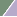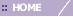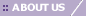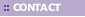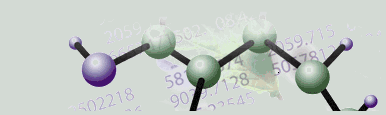# Glossary of Terms/Acronym List

Clicking the Index tab in the left pane of the online help may find additional information on terms not listed below

 A Annotations Comments or suggested links to additional information. Annotations are associated with items such as genes, samples, or datasets. Annotations editor The window that allows annotations to be viewed, added, modified and/or deleted. ANOVA or Analysis of Variance A statistical procedure to estimate the significance of differential expression between two or more groups of samples.  The test involves comparing the variance of the whole sample set to the variances within the groups – hence the name.  In GeneLinker the term ANOVA is used generically to describe both the F-test and the Kruskal-Wallis test.  (Some statistical texts use the term ANOVA for the F-test but not for the Kruskal-Wallis test.) Application The GeneLinker™ software. Apriori An association mining algorithm. Artificial Neural Network (ANN) A type of classifier (learner) loosely inspired by the interconnected nature of biological neurons. There are numerous excellent texts which discuss ANNs. Two are:  Christopher M. Bishop, Neural Networks for Pattern Recognition (Oxford: Clarendon/Oxford University Press, 1995), and Simon Haykin, Neural Networks:  A Comprehensive Foundation (New York: MacMillan, 1994). Association A pattern of feature values which occurs in a dataset more often than would be expected randomly. In GeneLinker™, a set of genes and their expression levels which co-occur with a certain sample class more often than would be expected randomly. Association mining The process of searching a dataset for associations. The algorithm used in GeneLinker™ Platinum is SLAM™. Attribute A single property of the dataset. B Bubble neighborhood A rectangular neighborhood around a node, where the bounds are based on the current radius. The left boundary is radius nodes to the left of the node (including the node itself). Similarly, the top, right and bottom boundaries are radius nodes up, to the right and down from the node respectively. A neighborhood with a radius of one contains only a single node. C Centroid Plot Useful for visualizing the centroid or exemplar points for each of the resulting clusters of a non-hierarchical experiment. Chebychev distance metric The maximum distance between two points X=(X1, X2, etc.) and Y=(Y1, Y2, etc.) along a single dimension. Classification (1) A division of a set of samples into classes; a discrete categorical variable. (2) The process of assigning or predicting the class of a sample. Classifier A device which assigns or predicts classes based on the pattern of features shown by a sample. For example, a classifier might be trained to predict whether a gene expression pattern arises from one cancer type or another. GeneLinker™ Platinum uses a committee of neural networks as a classifier. Clustering Also referred to as Cluster Analysis, this is a technique for sorting cases (genes, samples, etc.) into groups, or clusters, so that the degree of association is strong between members of the same cluster and weak between members of different clusters. Data subsets of genes or samples get grouped together (clustered) based on their similarities. Clustering techniques include Agglomerative Hierarchical, K-Means, Jarvis-Patrick and SOM. Cluster Plot Used to display the profiles of the individual members within a cluster. Color Matrix Plot A color plot used to visualize a dataset of values (e.g. gene expression levels). The display consists of a tiled grid of colored squares, samples in the rows, genes (note that gene names are case-sensitive) in the columns, and a legend. It can also be used to view a results of Principal Component Analysis. Comb A comb is a structure used in a Matrix Tree or Two Way Matrix Tree plot of a dataset that has a flat (non-hierarchical) cluster structure. The comb is analogous to the dendrogram which is used to show hierarchical structure. Committee of neural networks An ensemble of neural networks, each one of which is trained slightly differently, that together makes predictions. Component classifier A member of a committee of neural networks (see above). Also known as a learner. Continuous data, continuous variable A trait or variable which can assume any of a range of numerical values. For instance, gene expression data is continuous. Contrast 'discrete'. CSV file A Comma Separated Value file is a typical file type used for storing data. Each record is stored as text, a comma delimiter separates each field, and a line feed and a return character mark the end of the record. Cy5/Cy3 The ratio of two fluorescent intensities (Cy5 dye and Cy3 dye) on a spotted array. D Data mining Also known as Knowledge Discovery and Data mining (KDD). Data mining is an automated analysis process used for gleaning valid, previously unknown, potentially useful information from stored data. Data point A single item in a dataset. Each item has one value for each attribute (or feature) of the data space in which the dataset exists. Delimiter A separator between data values (see CSV File). Dendrograms A pictorial description of the hierarchy created through hierarchical clustering. It shows at a glance which clusters are strongly or weakly joined by indicating the distance between them when they were joined. See also Matrix Tree Plots and Partitional Clustering Plots. Contrast 'comb'. Discrete data, discrete variable A trait or variable which can only assume a small number of distinct values is said to be discrete. For instance, 'gender' is a discrete variable which can typically assume one of two values in humans. Contrast 'continuous'. Distance metrics Quantitative measurements of similarity between two data points under study. E EST 1. Eastern Standard Time 2. Expressed Sequence Tags, short segments of cDNA used to uniquely identify a gene. Euclidean distance metric The straight line distance between any two points. Exemplar A model attribute value derived from example of that attribute. This can be done statistically or by selecting a representative example. Exemplar point A data point with attribute values such that its attribute signature represents the attribute signature of the collection or data points it represents. Experiments navigator pane The hierarchical tree control for datasets and experiments. It is the upper left pane of the GeneLinker™ main window. The pane has three tabs (Experiments, Genes and Gene Lists). Experiments is the default. Expression level mRNA abundance, commonly measured by fluorescent intensities on gene chips. F Feature In machine learning, a trait used as input to supervised or unsupervised learning experiment. In GeneLinker™, genes are features. Feature Selection The process of deciding which available features a classifier will use as inputs. Filtering Methods that allow the exclusion of some genes from further analysis. Flat Classification Structure A classification structure in which no cluster contains any other cluster. See also Partitional Clustering. F-Test A parametric ANOVA intended to estimate the significance of differential expression between two or more groups of samples.  The F-test is designed for normally-distributed data and can give misleading results if applied to severely non-normal data. G GenBank A public repository of DNA, maintained by the NCBI (Website: http://www.ncbi.nlm.nih.gov/GenBank see Disclaimer). Gene Chip See Microarray. Gene expression The relative abundance of all mRNA species in a cell or tissue as they vary with environmental or biological factors or conditions. Gene Expression Profile Line plot showing how gene properties vary with environmental or biological factors or conditions. Globular Cluster A cluster which is very roughly spherical or elliptical is referred to as globular. A more precise mathematical term is convex, which roughly means that any line you can draw between two cluster members stays inside the boundaries of the cluster. Contrast 'non-globular cluster' - it may have a very complicated, convoluted boundary. Members of globular clusters typically bear some resemblance to the mean of the cluster. The mean of a non-globular cluster is often irrelevant, and can even lie outside the cluster. Green dye intensity The sample of interest, or denominator, in a spotted array relative gene expression ratio experiment. Also described as a Cy5/Cy3, test/background experiment, where in this case it represents Cy3 or background. H Hierarchical clustering A method of cluster analysis in which data is organized into a tree-like graph based on similarity. Agglomerative Hierarchical Clustering is a bottom up clustering method in which all data points start in individual clusters, and at each step of the clustering process the two closest clusters are merged until only one cluster remains. Divisive Hierarchical Clustering is a top-down clustering method and is essentially the reverse of agglomerative hierarchical clustering. GeneLinker™ does not support divisive hierarchical clustering. Housekeeping genes A housekeeping gene is a gene that is assumed to be constitutively expressed at a constant level. Common examples include beta-actin and GAPDH. Although they are assumed to be constitutive, they are often expressed at different levels and hence need to be normalized. Hybridization array An array where hybridization occurs between the pre-attached genetic materials (DNA, RNA etc.) and relevant complementary genetic materials (DNA, RNA etc.) under study. I Iteration (SOM) A single step within which the map 'learns' a single item from the input dataset. J Jarvis-Patrick clustering A clustering method; see Overview of Jarvis-Patrick Clustering for detailed information. K K-Means clustering An algorithm that generates fixed-sized, flat classifications and clusters based on distance metrics for similarity. The specified K value will determine the number of clusters that are created. See Overview of K-Means Clustering for detailed information. Kruskal-Wallis A non-parametric ANOVA intended to estimate the significance of differential expression between two or more groups of samples.  The Kruskal-Wallis test is applicable to any sort of data, whether normally-distributed or not, but is less powerful than the analogous F-test. L Linear Discriminant Analysis (LDA)A probabilistic classification model that produces linear boundaries between samples from different classes. Loadings Line Plot The Loadings Line Plot is one of three closely related plots (Loadings Line Plot, Loadings Scatter Plot, and Loadings Color Matrix Plot) that displays the individual elements of the PCs in Principal Component Analysis, allowing you too see the relative influence of genes or samples on the PCs. Loadings Scatter Plot The component loadings are the linear combinations for each principal component, and express the correlation between the original variables and the newly formed components. This type of scatter plot is used for PCA, where the x and y axes represent user-selected principal components. This shows the correlation of the variables with the user-selected principal components. Loadings Color Matrix Plot The loadings of a given PC represent the relative extent to which the original variables (genes or samples, depending on the Orientation selected for the PCA) influence the PC. The Loadings Color Matrix Plot displays these loadings as a tiled grid of colored rectangles such as those typically used to view tables and clustering results. Lowess Locally Weighted Regression and Smoothing Scatter plots. M Manhattan distance metric The distance between two points X=(X1, X2, etc.) and Y=(Y1, Y2, etc.) computed as the sum of the distances along every dimension. Map (SOM) A collection of interconnected nodes. Matrix Tree Plot A tree plot used to visualize clustering relationships for hierarchical clusterings; can also be used to represent partitional clusterings. See Dendrograms and Partitional Clustering. Matthews correlation Matthews correlation measures the predictive accuracy of an association for its class. If all samples in the dataset at labelled true positive,  true negative, false positive or false negative, and their frequencies represented by TP, TN, FP, FN then the Matthews correlation = (TP*TN-FP*FN)/sqrt[(TP+FP)*(TN+FN)*(TP+FN)*(FP+TN). Microarray A group of DNA features arranged on a microchip; may be high-density (i.e. more than 2500 features per chip) or low-density (2500 features or fewer per chip). Some researchers prefer to use high density microarrays which provide more information, some of it not required; others prefer to use customized low-density microarrays that contain only the data of interest. Microarray process The process of moving a sample from a source plate to the microarray, hybridizing the microarray with probes, scanning the slide, and evaluation of the spots. Example: collect the mRNA sample, isolate the nucleic acid, purify the products, deposit the DNA to create a microarray, hybridize a fluorescent probe to the microarray, detect the fluorescence using a scanner, and analyze the fluorescent image. PPSI Predictive Patterns Software Inc. N Navigator The upper left pane of the GeneLinker™ main window. Referred to as the Experiments, Genes or Gene Lists navigator pane, depending on which of the three tabs is selected. Experiments is the default. Neighborhood On a map, a node's neighborhood consists of all nodes that are in close proximity to it. Neighbors in Common Refers to the number of data points in the nearest neighbor list that two data points must have in common for the two data points to be clustered together. The Jarvis-Patrick clustering algorithm clusters two data points together if they are in each other's near neighbor list and have at least a minimum (specified) number of Neighbors in Common. Neighbors to Examine Refers to the minimum required number of near neighbors to examine for a particular data point. The Jarvis-Patrick clustering algorithm clusters two data points together if they are in each other's nearest neighbor list and have at least a minimum (specified) number of nearest Neighbors in Common. This value limits the number of nearest Neighbors to Examine when determining the number of Neighbors in Common. Neural network See Artificial Neural Network. N-Fold Culling A filtering method that allows genes without a large enough relative change to be ignored during analysis. Node (SOM) A single unit within a map. Non-globular clusters In contrast to globular clusters, non-globular clusters do not have well defined centers. Non-globular clusters can have a chainlike shape. Algorithms such as Jarvis-Patrick are good at finding chainlike clusters. Normality, normally-distributed Data which have a histogram with a particular bell-shape, also referred to as a Gaussian distribution, are normally-distributed.   See any basic statistical text for a detailed discussion.  You can examine a histogram of your data in GeneLinker using the Summary Statistics function. Normalization A family of techniques intended to ensure that all variables have equivalent status and all samples have equivalent status during analysis. This may involve adjustments to remove non-biological sources of variability, or to remove biological sources of variability which are known to be irrelevant to the scientific question at hand. O Outlier An outlier refers to a data point that exists outside the main grouping of data points. Outliers can be the result of experimental error or other environmental causes. Overtraining A common problem in supervised learning in which increasing accuracy on training data results, paradoxically, in decreasing accuracy on test data. P Partitional clustering Partitional clustering shows cluster membership by drawing a set of 'comb' structures, where each 'comb' connects entries in the same cluster. These plots visualize the results of partitional clustering algorithms (e.g. K-Means, Jarvis-Patrick). See also Dendrograms and Matrix Tree Plots. PC Principal Component PCA Principal Component Analysis, a method of projecting data onto a lower-dimensional subspace in a way that is optimal in a sum-squared error sense. Pearson Correlation A measurement of the linear dependencies between two variables. Preprocessing The act of arranging data so that it is in an acceptable format for optimal use in a software application. P-Value The probability that a given effect is due to random chance as opposed to a systematic influence. More precisely, the p-value is the probability of observing the data or observing the effect when a null hypothesis is true, the null hypothesis asserting that there is no systematic influence. The observed effect, for example, might be the difference between the expression of a certain gene under a treatment condition and its expression under a different condition. A p-value must fall between 1 and zero. A p-value near one implies an observed effect that can easily occur by chance (i.e., an insignificant effect). Whereas, a p-value near zero (e.g., 0.01 or smaller) implies little role for chance to account for the observed effect (i.e., a statistically significant effect due to some kind of systematic influence). Q Quadratic Discriminant Analysis (QDA)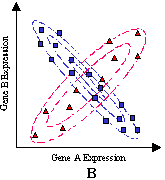A probabilistic classification model that produces non-linear, curved boundaries between samples from different classes. R Radius length (SOM) The distance, counted in nodes, over which a new cluster item's influence is felt during learning. Random Seed The random seed allows you to always get identical results when you repeat any type of analysis that uses a random number generator (e.g. the initial random assignment of points in K-means clustering, or the random sampling of rows in SLAM). Since computers are deterministic, they don't really generate random numbers. They use pseudo random number generators to mimic random numbers. A pseudo random number generator is essentially a function that produces a sequence of numbers that appear random. The actual pseudo random number generator takes the current number in a sequence and produces the next number in the sequence. The random seed is essentially a way of specifying exactly where to start in this sequence. If you specify the same random seed, you will always get the same behaviour if you try to repeat an analysis. If you specify a different random seed, you will probably get slightly different results. You might be able to get a sense of how robust your results are if you tend to see the same results with different random seeds. Record In a comma-delimited file (.csv) a record is a row of data. A record generally refers to a sample as samples are usually in the rows of a dataset. Red dye intensity The sample of interest, or numerator, in a spotted array relative gene expression ratio experiment. Also described as a Cy5/Cy3, test/background experiment, where in this case it represents Cy5 or test. Reference vector (SOM) A sequence of feature values. The reference vector is comparable to (i.e. has the same dimensions as) items to be clustered. Representative variable The designated key variable in training a classifier or running SLAM™. Typically this will be the variable which you are trying to predict, e.g. tissue type or disease class. Contrast 'feature'. Robust A classifier which makes accurate predictions on test data is said to be robust. S Sample All gene expression measurements from a single hybridization or chip or microarray experiment. A single row in GeneLinker (usually). Scaling Adjusting the values across samples (gene chips) so that the slope of each sample is equivalent. Scatter Plot A summary of the data showing the relationship between two variables (represented by X and Y axes). Score Plot The component scores are the data on the principal components. They project the original individuals onto the newly formed components, and currently support 2D and 3D score plots. The Score Plot is a scatter plot used for PCA, where the axes represent user-selected principal components. The plot contains the individuals projected onto those principal components. Scree Plot A simple line or bar plot for PCA; shows the ordered percentage of variance explained by each principal component. It resembles a scree slope (where rocks have fallen down the side of a mountain). Session The time span between starting (opening) and stopping (closing, exiting) the GeneLinker™ application. SLAM™ An acronym for Sub-Linear Association Mining, SLAM™ is PPSI's proprietary fast stochastic method for association mining in discrete data. SOM (Self Organizing Map) A SOM is an algorithm that forms a topologically ordered mapping from the input signal space onto a neural network. It can be thought of as a non-linear projection of the probability density function of the input signal space onto a two-dimensional map. It organizes a set of samples on a map such that their distribution indicates their relative similarities. SOMs can be used for preprocessing patterns for their recognition, or, if the neural network is a regular two-dimensional array, to project and visualize high-dimensional signal spaces on such a two dimensional display. Spearman Correlation A measure that identifies certain linear and non-linear correlations between sequences. Spearman Correlation ranks the values of two sequences and finds the linear correlation of the ranks. Spotted array A microarray of genes (printed by a robot, usually spot cDNA) containing many features (spots), where each spot corresponds to a specific gene. Therefore, the intensity of the spots on the array indicates where more information is present for a specific gene. Spotted array scaling The process of taking the multiple measurements taken for each gene and reducing them to a single value less biased or more representative than the constituent measurements if taken alone. The most common case will involve measuring Cy5 and Cy3 fluorescent intensity values and calculating their ratio. The process can also include background measurements for Cy5 and Cy3, subtracting their values before calculating the ratio. Statistic Used to rank associations (all and within a class) in terms of their relevance to the target variable (Matthews column, phenotype, potential consequent). Status bar The bar that appears in the lower right corner of the application used to display information to the user. Stochastic Describes any algorithm which employs random sampling and therefore may show some variation in results when run over and over again on the same data. Sub-experiment An experiment derived from another experiment. Supervised analysis, Supervised learning Supervised analysis finds patterns in high-dimensional data by initially relying upon some assumptions of particular categories or relationships in the data. Commonly used techniques include classifiers such as linear discriminants, artificial neural networks, and support vector machines. These have been successfully applied to many different kinds of data. For gene expression data, these methods are often used to assign an observed expression profile to a predetermined class. Support In association mining, the number of samples in a dataset in which a given association appears. SVM Support Vector Machine. Algorithm used to identify patterns in datasets. T Tab-delimited A data file which uses the tab character (ASCII character 9) to separate entries within a row. Tabular A data file in the form of a regular table is described as tabular.  Each line of a tabular data file has the same number of fields (or columns, or delimiters).  Each row corresponds to a sample and each column to a gene, or vice versa. Target node (SOM) The node in the map that is most similar to the selected item from the input dataset. Target variable See Representative variable. Test data Data held back from a classifier until after it is trained. The classifier is then used to make predictions about the test data. The accuracy of those predictions is a fair measure of the accuracy that the classifier can be expected to make on any similar data in the future. Training A classifier must be exposed to known samples before it can be used to make predictions on unknown samples. This process of optimizing the classifier's internal parameters is called training. Training data Data used as examples to train a classifier. Training samples must have known classes associated with them. These known classes comprise the representative variable for training. Transformation A technique to achieve a different dataset by applying some user-defined functions to the original data. U Uniform/Gaussian Discriminant Analysis (UGDA)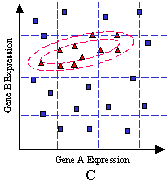A probabilistic classification model that treats one class as a diffuse ‘background’ class, and the other classes as ‘hot spots’, defined by elliptical boundaries. Unsupervised analysis, Unsupervised learning Unsupervised analysis finds patterns in high-dimensional data without relying upon a priori assumptions of particular categories or relationships in the data. Techniques include hierarchical clustering, K-Means clustering, and Self-Organizing Maps (SOM). These have been successfully applied to a wide variety of complex data including microarrays. V Validation data Data used to validate or control the training of a classifier. Variable In GeneLinker™, a set of observations associated with samples. For instance, if a pathologist determined a tumor type for each sample in a dataset those observations might comprise a variable named 'known tumor type'. Such a variable could be compared against other variables of the same type (see below), e.g. 'predicted tumor type'. Variable type Variables which comprise distinct measurements of the same phenomenon are grouped together in GeneLinker™ into variable types. An example of a  variable type is 'tumor type', and two variables of that type might be 'known' and 'predicted by model #4'. Vector Mathematically, this is a sequence of numbers; biologically, this is an agent that transfers material (usually DNA). Visualization A method used to view gene expression data profiles using tables or graphs (e.g. Scatter Plots, Matrix Tree Plots, Color Matrix Plots, etc.). W X XML eXtensible Markup Language Y Z